## Scalar Triple Product

Scalar triple product is a multiplication of three vectors that produces a scalar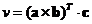Example
Suppose we have three vectors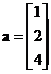,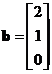and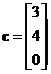.

First we compute cross productThen, the scalar triple product is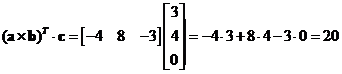Geometrically, scalar triple product is equal to the volume of parallelepiped. The based area of the parallelepiped is a parallelogram whose area is equal to the absolute value of the cross product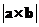.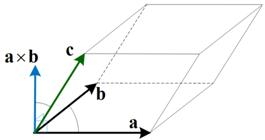The interactive program below helps you to compute Scalar triple product algebraically. You input 3 vectors of the same dimension. The program output is the scalar triple product. Random example button will generate random vectors at the right format.

(a * b ) . c

## Properties

Some important properties of related to scalar triple product are

• Scalar triple product is invariant under cyclic permutation of the vectors, that is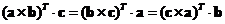• Scalar triple product is zero if two of the vectors lie on the same plane or are parallel to the same plane (i.e. coplanar)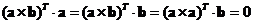.
• Three vectors are linearly dependent (coplanar) if and only if their scalar triple product is zero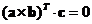.
• Three vectors form a basis if and only if their scalar triple product is not zero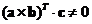. The basis is right handed if the scalar triple product is positive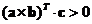and called left handed if the scalar triple product is negative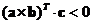.